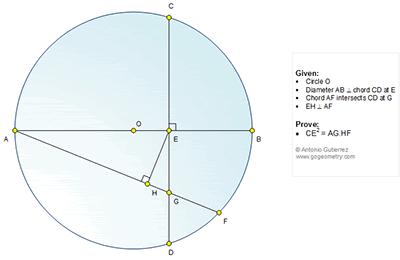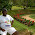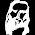## Thursday, October 8, 2015

### Geometry Problem 1151: Circle, Diameter, Chord, Perpendiculars, 90 Degrees, Metric Relations

Geometry Problem. Post your solution in the comments box below.
Level: Mathematics Education, High School, Honors Geometry, College.

Click the diagram below for more details.#### 3 comments:

1.Let the radius be R and let OE = s
Let AG = a. GF = b and GH = c

From similar Tr.s

(R+s)(R-s) = CE^2 = ED^2. (1)

(R+s)(2R) = a(a+b). (2)
(R+s)^2 = a(a-c). (3)

(2) - (3)
(R+s)(R-s) = a(a+b) - a(a-c) = a(b+c) which from (1) equals CE ^2 which is the required result.

Sumith Peiris
Moratuwa
Sri Lanka

2.Since BEGF concyclic, thus

CE² = AE×EB
= AE×AB - AE²
= AG×AF - AG×AH
= AG×HF

3.For (1) I've used similar Tr.s AED & BED, for (2) Tr.s ABF and AEG and for (3) Tr.s AEH & AEG